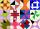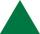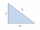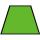# Isosceles triangle calculator - result

Please enter two properties of the isosceles triangle

Use symbols: a,b c, h, T, p, A, B, C, r, R

You have entered side b and perimeter o.

### Acute isosceles triangle.

Sides: a = 44   b = 44   c = 29

Area: T = 602.361113545
Perimeter: p = 117
Semiperimeter: s = 58.5

Angle ∠ A = α = 70.75988112369° = 70°45'32″ = 1.23549742309 rad
Angle ∠ B = β = 70.75988112369° = 70°45'32″ = 1.23549742309 rad
Angle ∠ C = γ = 38.48223775263° = 38°28'57″ = 0.67216441918 rad

Height: ha = 27.38800516113
Height: hb = 27.38800516113
Height: hc = 41.54221472724

Median: ma = 30.07549064836
Median: mb = 30.07549064836
Median: mc = 41.54221472724

Vertex coordinates: A[29; 0] B[0; 0] C[14.5; 41.54221472724]
Centroid: CG[14.5; 13.84773824241]
Coordinates of the circumscribed circle: U[14.5; 18.24105111376]
Coordinates of the inscribed circle: I[14.5; 10.29767715461]

Exterior (or external, outer) angles of the triangle:
∠ A' = α' = 109.2411188763° = 109°14'28″ = 1.23549742309 rad
∠ B' = β' = 109.2411188763° = 109°14'28″ = 1.23549742309 rad
∠ C' = γ' = 141.5187622474° = 141°31'3″ = 0.67216441918 rad

# How did we calculate this triangle?

The calculation of the triangle progress in two phases. The first phase is such that we try to calculate all three sides of the triangle from the input parameters. The first phase is different for the different triangles query entered. The second phase is the calculation of other characteristics of the triangle, such as angles, area, perimeter, heights, the center of gravity, circle radii, etc. Some input data also results in two to three correct triangle solutions (e.g., if the specified triangle area and two sides - typically resulting in both acute and obtuse) triangle).

### 2. From side b we calculate side a:

$a = b = 44$

### 3. From perimeter o and side a we calculate side c:

$o = 2a + c \ \\ c = o - 2a = 117 - 2 \cdot \ 44 = 29$

Now we know the lengths of all three sides of the triangle, and the triangle is uniquely determined. Next, we calculate another its characteristics - same procedure as calculation of the triangle from the known three sides SSS.

$a = 44 \ \\ b = 44 \ \\ c = 29$

### 4. The triangle perimeter is the sum of the lengths of its three sides

$p = a+b+c = 44+44+29 = 117$

### 5. Semiperimeter of the triangle

The semiperimeter of the triangle is half its perimeter. The semiperimeter frequently appears in formulas for triangles that it is given a separate name. By the triangle inequality, the longest side length of a triangle is less than the semiperimeter.

### 6. The triangle area using Heron's formula

Heron's formula gives the area of a triangle when the length of all three sides are known. There is no need to calculate angles or other distances in the triangle first. Heron's formula works equally well in all cases and types of triangles.

$T = \sqrt{ s(s-a)(s-b)(s-c) } \ \\ T = \sqrt{ 58.5(58.5-44)(58.5-44)(58.5-29) } \ \\ T = \sqrt{ 362838.94 } = 602.36$

### 7. Calculate the heights of the triangle from its area.

There are many ways to find the height of the triangle. The easiest way is from the area and base length. The area of a triangle is half of the product of the length of the base and the height. Every side of the triangle can be a base; there are three bases and three heights (altitudes). Triangle height is the perpendicular line segment from a vertex to a line containing the base.

$T = \dfrac{ a h _a }{ 2 } \ \\ \ \\ h _a = \dfrac{ 2 \ T }{ a } = \dfrac{ 2 \cdot \ 602.36 }{ 44 } = 27.38 \ \\ h _b = \dfrac{ 2 \ T }{ b } = \dfrac{ 2 \cdot \ 602.36 }{ 44 } = 27.38 \ \\ h _c = \dfrac{ 2 \ T }{ c } = \dfrac{ 2 \cdot \ 602.36 }{ 29 } = 41.54$

### 8. Calculation of the inner angles of the triangle using a Law of Cosines

The Law of Cosines is useful for finding the angles of a triangle when we know all three sides. The cosine rule, also known as the law of cosines, relates all three sides of a triangle with an angle of a triangle. The Law of Cosines is the extrapolation of the Pythagorean theorem for any triangle. Pythagorean theorem works only in a right triangle. Pythagorean theorem is a special case of the Law of Cosines and can be derived from it because the cosine of 90° is 0. It is best to find the angle opposite the longest side first. With the Law of Cosines, there is also no problem with obtuse angles as with the Law of Sines, because cosine function is negative for obtuse angles, zero for right, and positive for acute angles. We also use inverse cosine called arccosine to determine the angle from cosine value.

$a^2 = b^2+c^2 - 2bc \cos α \ \\ \ \\ α = \arccos(\dfrac{ b^2+c^2-a^2 }{ 2bc } ) = \arccos(\dfrac{ 44^2+29^2-44^2 }{ 2 \cdot \ 44 \cdot \ 29 } ) = 70^\circ 45'32" \ \\ \ \\ b^2 = a^2+c^2 - 2ac \cos β \ \\ β = \arccos(\dfrac{ a^2+c^2-b^2 }{ 2ac } ) = \arccos(\dfrac{ 44^2+29^2-44^2 }{ 2 \cdot \ 44 \cdot \ 29 } ) = 70^\circ 45'32" \ \\ γ = 180^\circ - α - β = 180^\circ - 70^\circ 45'32" - 70^\circ 45'32" = 38^\circ 28'57"$

An incircle of a triangle is a circle which is tangent to each side. An incircle center is called incenter and has a radius named inradius. All triangles have an incenter, and it always lies inside the triangle. The incenter is the intersection of the three angle bisectors. The product of the inradius and semiperimeter (half the perimeter) of a triangle is its area.

$T = rs \ \\ r = \dfrac{ T }{ s } = \dfrac{ 602.36 }{ 58.5 } = 10.3$

The circumcircle of a triangle is a circle that passes through all of the triangle's vertices, and the circumradius of a triangle is the radius of the triangle's circumcircle. Circumcenter (center of circumcircle) is the point where the perpendicular bisectors of a triangle intersect.

$R = \dfrac{ a b c }{ 4 \ r s } = \dfrac{ 44 \cdot \ 44 \cdot \ 29 }{ 4 \cdot \ 10.297 \cdot \ 58.5 } = 23.3$

### 11. Calculation of medians

A median of a triangle is a line segment joining a vertex to the midpoint of the opposite side. Every triangle has three medians, and they all intersect each other at the triangle's centroid. The centroid divides each median into parts in the ratio 2:1, with the centroid being twice as close to the midpoint of a side as it is to the opposite vertex. We use Apollonius's theorem to calculate the length of a median from the lengths of its side.

$m_a = \dfrac{ \sqrt{ 2b^2+2c^2 - a^2 } }{ 2 } = \dfrac{ \sqrt{ 2 \cdot \ 44^2+2 \cdot \ 29^2 - 44^2 } }{ 2 } = 30.075 \ \\ m_b = \dfrac{ \sqrt{ 2c^2+2a^2 - b^2 } }{ 2 } = \dfrac{ \sqrt{ 2 \cdot \ 29^2+2 \cdot \ 44^2 - 44^2 } }{ 2 } = 30.075 \ \\ m_c = \dfrac{ \sqrt{ 2a^2+2b^2 - c^2 } }{ 2 } = \dfrac{ \sqrt{ 2 \cdot \ 44^2+2 \cdot \ 44^2 - 29^2 } }{ 2 } = 41.542$

### An isosceles triangle in word problems in mathematics:

• Isosceles triangleCalculate the perimeter of isosceles triangle with arm length 73 cm and base length of 48 cm.
• Isosceles triangleCalculate the area of an isosceles triangle, the base of which measures 16 cm and the arms 10 cm.
• Isosceles IVIn an isosceles triangle ABC is |AC| = |BC| = 13 and |AB| = 10. Calculate the radius of the inscribed (r) and described (R) circle.
• QuizQAn isosceles triangle has two sides of length 7 km and 39 km. How long is a third side?
• Length ITFind the length (circumference) of an isosceles trapezoid in which the length of the bases a,c and the height h are given: a = 8 cm c = 2 cm h = 4 cm
• Isosceles right triangleCalculate the area of an isosceles right triangle whose perimeter is 377 cm.
• Isosceles IIIThe base of the isosceles triangle is 17 cm area 416 cm2. Calculate the perimeter of this triangle.
• Isosceles right triangleContents of an isosceles right triangle is 18 dm2. Calculate the length of its base.
• Arm-legCalculate the length of the base of an isosceles triangle with a circumference 224 cm if the arm length is 68 cm.
• Angle at the apexIn an isosceles triangle, the angle at the apex is 30° greater than the angle at the base. How big are the internal angles?
• IS trapezoidIsosceles trapezoid arm measured 35 cm. Height is 30 cm and middle segment is 65 cm. Determine length of its bases.
• Isosceles triangleWhat are the angles of an isosceles triangle ABC if its base is long a=5 m and has an arm b=4 m.
• Isosceles trapeziumCalculate the area of an isosceles trapezium ABCD if a = 10cm, b = 5cm, c = 4cm.
• Medians of isosceles triangleThe isosceles triangle has a base ABC |AB| = 16 cm and 10 cm long arm. What are the length of medians?
• Isosceles trapezoidCalculate the area of an isosceles trapezoid whose bases are in the ratio of 4:3; leg b = 13 cm and height = 12 cm.